Search

Introduction to Matrices / Matrix Size (page 1 of 3)

Sections: Augmented & coefficient matrices / Matrix size, Matrix notation & types, Matrix equalityAugmented matrices

Matrices are incredibly useful things that crop up in many different applied areas. For now, you'll probably only do some elementary manipulations with matrices, and then you'll move on to the next topic. But you should not be surprised to encounter matrices again in, say, physics or engineering. (The plural "matrices" is pronounced as "MAY-truh-seez".)

Matrices were initially based on systems of linear equations.

• Given the following system of equations, write the associated augmented matrix.
• 2x + 3y  z = 6
x  y  z = 9
x + y + 6z = 0

Write down the coefficients and the answer values, including all "minus" signs. If there is "no" coefficient, then the coefficient is "1".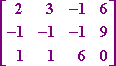That is, given a system of (linear) equations, you can relate to it the matrix (the grid of numbers inside the brackets) which contains only the coefficients of the linear system. This is called "an augmented matrix": the grid containing the coefficients from the left-hand side of each equation has been "augmented" with the answers from the right-hand side of each equation.

The entries of (that is, the values in) the matrix correspond to the x-, y- and z-values in the original system, as long as the original system is arranged properly in the first place. Sometimes, you'll need to rearrange terms or insert zeroes as place-holders in your matrix.

• Given the following system of equations, write the associated augmented matrix.

x + y = 0
y + z = 3

z  x = 2

I first need to rearrange the system as:

x + y       = 0
y + z = 3

x      + z = 2

Then I can write the associated matrix as:When forming the augmented matrix, use a zero for any entry where the corresponding spot in the system of linear equations is blank.

Coefficient matrices

If you form the matrix only from the coefficient values, the matrix would look like this:Above, we went from a linear system to an augmented matrix. You can go the other way, too.

• Given the following augmented matrix, write the associated linear system.
•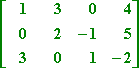Remember that matrices require that the variables be all lined up nice and neat. And it is customary, when you have three variables, to use x, y, and z, in that order. So the associated linear system must be:

x + 3y       =  4
2y  z =  5

3x       + z = 2

The Size of a matrix

Matrices are often referred to by their sizes. The size of a matrix is given in the form of a dimension, much as a room might be referred to as "a ten-by-twelve room". The dimensions for a matrix are the rows and columns, rather than the width and length. For instance, consider the following matrix A: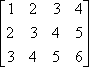Since A has three rows and four columns, the size of A is 3 × 4 (pronounced as "three-by-four").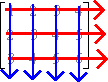The rows go side to side; the columns go up and down. "Row" and "column" are technical terms, and are not interchangable. Matrix dimensions are always given with the number of rows first, followed by the number of columns. Following this convention, the following matrix B: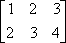...is 2 × 3. If the matrix has the same number of rows as columns, the matrix is said to be a "square" matrix. For instance, the coefficient matrix from above:...is a 3 × 3 square matrix.

Top  |  1 | 2 | 3  |  Return to Index  Next >>

 Cite this article as: Stapel, Elizabeth. "Introduction to Matrices / Matrix Size." Purplemath. Available from     https://www.purplemath.com/modules/matrices.htm. Accessed [Date] [Month] 2016

Study Skills Survey

Tutoring from Purplemath
Find a local math tutor